﻿ 基于SPI指数的近54年豫中地区干旱时空演变特征

# 基于SPI指数的近54年豫中地区干旱时空演变特征Evolution Characteristics of Drought in the Region of Henan Province in Recent 54 Years Based on SPI

Abstract: Drought is in a long enough period of time, serious shortage of rainfall, resulting in soil due to evaporation and moisture loss, a decrease in streamflows, undermining the normal growth of crops and human activities of disastrous weather phenomenon. Based on 1961-2014 of monthly mean temperature and precipitation data, using the standardized precipitation index (SPI) quan-titative analysis of the drought in central Henan Province, monthly scale, seasonal scale, scale for the first half of the year and annual scales on the frequency of occurrence are analyzed, which reveals the time evolution characteristics of the drought and the causes of drought occurrence. The results show that SPI value can better reflect the spatial and temporal characteristics of drought in the region of Henan Province. With the increase of time scale, the fluctuation range of SPI value decreases, and the frequency of drought decreases. In the past 54 years, drought occurred in half a year, season and month scale, the frequency of drought is on the rise, and the distribution between regions is not uniform; seasonal scale in autumn season drought occurred the most serious. During the inter annual changes of drought, the drought occurred in 2013, 1986-1988 and 1968 in the middle of Henan Province. The contribution of the Eurasian atmospheric circulation anomalies, the El Nino phenomenon and the rise of temperature to the occurrence of drought can not be ignored.

1. 引言

2. 研究区概况

3. 资料来源与研究方法

3.1. 资料来源

3.2. 研究方法

3.2.1. SPI指数

Mckee于1993年提出，由于不同时间、不同地区降水量变化幅度很大，直接用降水量很难在不同时空尺度上相互比较，而且降水分布是一种偏态分布，不是正态分布，所以在降水分析中，采用г分布概率来描述降水量的变化，然后再经正态标准化求得SPI值。其计算步骤如下 ：

1) 假设某时段降水量为随机变量x，则其г分布的概率密度函数为：

$f\left(x\right)=\frac{1}{{\beta }^{\gamma }\Gamma \left(\gamma \right)}{x}^{\gamma -1}{\text{e}}^{-x/\beta },x>0$ (1)

$\Gamma \left(\gamma \right)={\int }_{0}^{\infty }{x}^{\gamma -1}{\text{e}}^{-x}\text{d}x$ (2)

$\stackrel{^}{\gamma }=\frac{1+\sqrt{1+4A/3}}{4A}$ (3)

$\stackrel{^}{\beta }=\stackrel{¯}{x}/\stackrel{^}{\gamma }$ (4)

$A=\mathrm{lg}\stackrel{¯}{x}-\frac{1}{n}\underset{i=1}{\overset{n}{\sum }}\mathrm{lg}{x}_{i}$ (5)

$P\left(x<{x}_{0}\right)={\int }_{0}^{\infty }f\left(x\right)\text{d}x$ (6)

2) 降水量为0时的事件概率由下式估计：

$P\left(x=0\right)=m/n$ (7)

3) 对г分布概率进行正太标准化处理，即将(6)、(7)式求得的概率值代入标准化正态分布函数，即：

$P\left(x<{x}_{0}\right)=\frac{1}{\sqrt{2\text{π}}}{\int }_{0}^{\infty }{\text{e}}^{-{Z}^{2}/2}\text{d}x$ (8)

$Z=S\frac{t-\left({c}_{2}t+{c}_{1}\right)t+{c}_{0}}{\left(\left({d}_{3}t+{d}_{2}\right)t+{d}_{1}\right)t+1.0}$ (9)

${c}_{0}=2.515517$ , ${c}_{1}=0.802853$ , ${c}_{2}=0.010328$ ,

${d}_{1}=1.432788$ , ${d}_{2}=0.189269$ , ${d}_{3}=0.001308$ .

3.2.2. 干旱评价指标

1) 干旱频率(Pi)：根据某站点干旱发生年数计算相应的干旱发生频率，计算公式为：

${P}_{i}=n/N×100%$ (10)

2) 干旱强度(Sij)：用来评价干旱的严重程度，某个气象站某时段内的干旱强度可通过SPI值反应，计算公式：

${S}_{ij}=|\frac{1}{m}\underset{i=1}{\overset{m}{\sum }}SPIi|$ (11)Table 1. Drought grading of standardized rainfall index 

4. 结果与分析

4.1. 不同时间尺度SPI值表征的干旱特征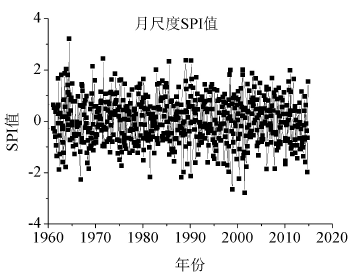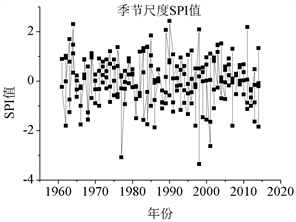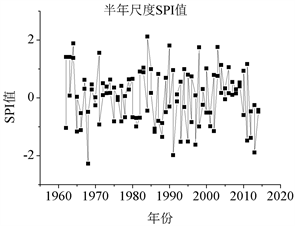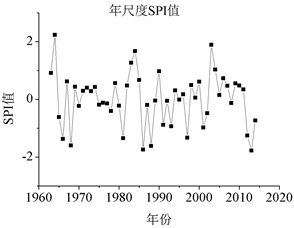Figure 1. 1961-2014 SPI values for different time scales in center region

1961~2014年豫中地区不同时间尺度历年SPI均值如图2所示。其中，年均SPI-12波动幅度最大，年均SPI-6次之，年均SPI-3变化较为平稳，年均SPI-1最为稳定，即随着时间尺度的增大年均SPI值波动幅度增大。SPI-12反映出的干旱情况与豫中地区历史上发生干旱的时间具有相对一致性，如豫中地区发生干旱的典型年份有1966年(中旱)，1968年(重旱)、1986年(重旱)、1988年(重旱)、1997年(中旱)，2013年(重旱)等。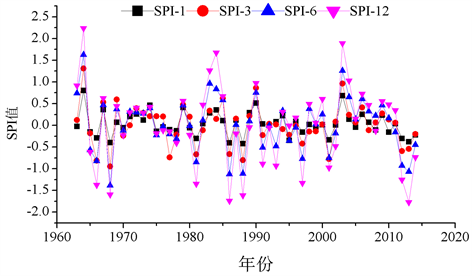Figure 2. 1961-2014 SPI mean for different time scales in center region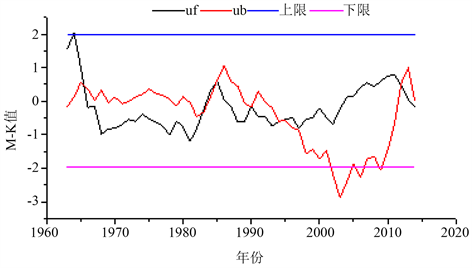Figure 3. M-K test of average SPI-12 sequence in center area in 1961-2014

4.2. 豫中地区干旱空间分布特征Table 2. Frequency of monthly drought occurrence in center areaTable 3. Frequency of drought occurrence at seasonal scale in center areaTable 4. Frequency of drought occurrence in half-scale in center areaTable 5. Frequency of annual drought occurrence in center area

5. 结论

1) SPI值变化趋势：SPI值的波动幅度随时间尺度的增大呈逐渐减小的趋势。即月尺度波动幅度最大，年尺度波动幅度最小。年均SPI值波动幅度随时间尺度的增大而呈递增的趋势。

2) 干旱发生的时间分布特征：就月尺度而言，SPI值变化幅度最大；季节尺度SPI值波动幅度较月尺度SPI值波动幅度平缓，较好的反映出干湿季变化的干旱的影响，其中，1976~1977年、1997~1998年波动幅度最大；半年尺度SPI值波动周期较季节尺度稍长，其中，1967~1968年和1990~1991年波动幅度较大；年尺度SPI值波动幅度最为稳定，可反映出干旱的年际变化特征，1966~1968年、1986~1988、2013年SPI值波动幅度最大。

3) 干旱发生的空间分布特征：豫中地区与豫北相比，干旱发生强度要小；与豫南相比，干旱发生强度要大，整体而言，豫中干旱发生强度频率较其他区域要小，相对不易发生干旱。区域内部，许昌干旱发生强度大于郑州，即由北向南干旱发生强度呈递增趋势。

6. 讨论

 IPCC (2013) Working Group I Contribution to the IPCC Fifth Assessment Report, Climate Change, 2013: The Physical Science Basis: Summary of for Policymakers.

 IPCC (2007) Climate Change 2007: The Physical Science Basis, Summary for Policy Makers. IPCC WGI Fouth Report, Paris, 195-199.

 袁文平, 周广胜. 干旱指标的理论分析与研究展望[J]. 地球科学进展, 2004, 19(6): 982-991.

 王劲松, 郭江勇, 周跃武, 等. 干旱指标研究的进展与展望[J]. 干旱区地理, 2007, 30(1): 60-65.

 姚玉璧, 董安详, 王毅荣. 基于帕默尔干旱指数的中国春季区域干旱特征比较研究[J]. 干旱区地理, 2007, 30(1): 22-29.

 张强. 华北地区干旱指数的确定及其应用[J]. 灾害学, 1998, 13(4): 34-38.

 翟禄新, 冯起. 基于SPI的西北地区气候干湿变化[J]. 自然资源学报, 2011, 26(5): 847-857.

 邹旭凯, 任国玉, 张强, 等. 基于综合气象干旱指数的中国干旱变化趋势研究[J]. 气候与环境研究, 2010, 15(4): 371-378.

 马柱国. 中国北方干旱区地表湿润状况的趋势分析[J]. 气象学报, 2001, 59(6): 737-746.

 袁文平, 周广胜. 标准化降水指数与Z指数在我国应用的对比分析[J]. 植物生态学报, 2004, 28(4): 523-529.

 周扬, 李宁, 吉中会, 等. 基于SPI指数的1981-2010年内蒙古地区干旱时空分布特征[J]. 自然资源学报, 2013, 28(10): 1694-1705.

 张竟竟, 郭志富, 李治国. 河南水旱灾害危险性时空特征研究[J]. 自然资源学, 2013, 28(6): 957-968.

 史本林, 朱新玉, 李宏忠, 等. 中原腹地气候变化对冬小麦产量的影响——以商丘为例[J]. 地理研究, 2012, 31(1): 14-22.

 吴燕锋. 北疆近52年气象干旱时空演变特征[D]: [硕士学位论文]. 乌鲁木齐: 新疆农业大学, 2016.

 刘晓静, 温馨. 基于SPI指数的近50年辽宁省干旱时空演变规律分析[J]. 吉林师范大学学报(自然科学版), 2016, 37(2): 146-151.

 Oliveira-Júnior, J.F.D., Gois, G.D., Terassi, P.M.D.B., et al. (2018) Drought Severity Based on the SPI Index and Its Relation to the ENSO and PDO Climatic Variability Modes in the Regions North and Northwest of the State of Rio de Janeiro-Brazil. Atmospheric Research, 212, 91-105.
https://doi.org/10.1016/j.atmosres.2018.04.022

Top